# 鉸鏈波紋補償器(JY)型號示例

0317-8185517 8184517

1、確定位移量：

△X=al△T=13.2×10ˉ6(80+80)×103×(120-(-10°))=275mm

2、選用補償器：

LⅠ=1.1m,KθⅠ=197N·m

LⅡ=LⅢ=0.9m，KθⅡ=KθⅢ=295N·m/度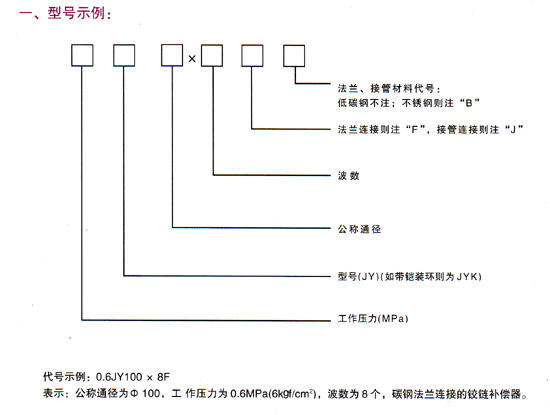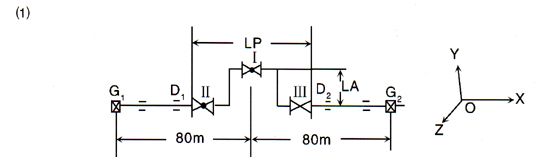（Lp為補償段安裝長度）

LA=1200 （在安裝跨距要求允許條件下，LA適當大一點好）

3、考慮冷緊問題：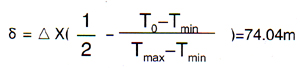4、計算支座承受載荷：

a、膨脹節變形力矩。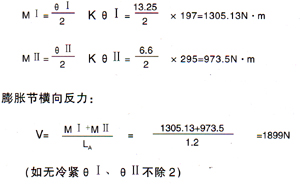b、 G1點：　　　　　　　　　　　　　　　　　　　D1點：

Fy=Fz=0 　　　　　　　　　　　　　　　　　　　　 Fx=Fy=Fz=0，Mx=My=0

Fx=-V=-1899N 　　　　　　　　　　　　　　　　　　Mz=MⅡ=973.5N·m

Mx=My=Mz=0

G2：

Fx=V=1899N 　　　　　　　　　　　　　　　　　D2：Fx=Fy=Fz=0，Mx=My=0

Fy=Fz=0，Mx=My=Mz=0 　　　　　　　　　　　　　　Mz=-MⅡ=-973.5N·m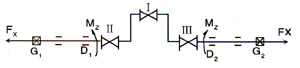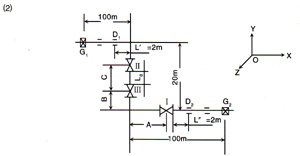1、確定位移：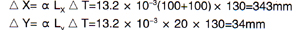2、選用補償器：

LⅠ=0.8m，KθⅠ=590N·m/度

LⅡ=LⅢ=1.1m，KθⅡ=197N·m/度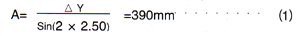（1）取大一點，如A=2m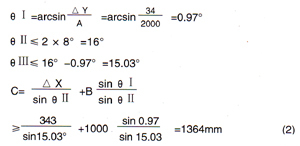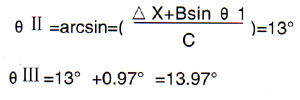3、考慮冷緊問題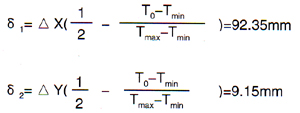4、計算支座承受載荷：

a、膨脹節變形力矩：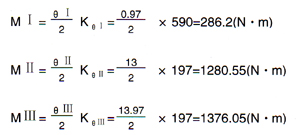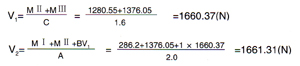b、G1點：

Fx=-V1=-1660.37N

Fy=Fz=0

Mx=My=Mz=0

D1點：

Fx=Fz=0，Mx=My=0

Fy=V2=1661.31N

M2=-MⅡ+V2×L′-V1（20-B-C）

=-1280.55+1661.31×2-1660.37(20-1.0-1.6)

=-26848.37(N·m)

D2點：

Fx=Fz=0，Mx=My=0

Fy=-V2=-1661.31(N)

Mz=MⅠ-Fy(2+LⅠ/2）=286.2+1661.31（2+0.8/2）=4273.34(Nm)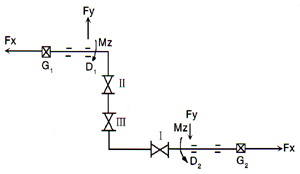QQ:2496772192 網址:http://www.chinaitlabs.net 郵箱：hbbtbwg@163.com

PowerBy：速貝·網搜寶 網站建設：中科四方“掃一掃”加入我們